# HW#13 - Quadratic Equation Solver

Write a spreadsheet that solves the quadratic equation.  Recall that a quadratic equation is a polynomial of the form:

ax2 + bx + c = 0

where a, b, and c are real constants.  The "roots" of a quadratic equation are real or complex values of x that satisfy the equation (when you plug in x with the selected values of a, b, and c, the equation evaluates to zero).

The calculations and decision making should all be performed in VB.  The user should enter the coefficients a, b, & c and then select a Solve button.  You should then read the values of a, b, & c and then display the results.  For example, your spreadsheet may look something like this: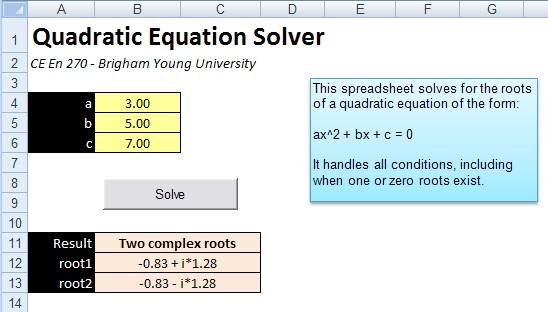When a is not equal to zero, the roots are given by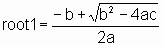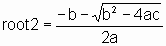The expression under the square root sign (b2 - 4ac) is the discriminant.

If the discriminant is positive, then two real roots exist.

If the discriminant is zero, then the two roots are real and equal.  In this case we say that the polynomial has one real root.

If the discriminant is negative, then the roots are complex.

When both a = 0 and b = 0, we consider the case extremely degenerate and leave it at that (no roots).

When a = 0 and b is not = 0, we consider the case degenerate.  In this case the equation reduces to

bx + c = 0

and it has one root given by x = -c / b.

For each set of values for a, b, and c your spreadsheet should print the computed root(s) along with one of the following messages:

extremely degenerate
degenerate
one real root
two real roots
two complex roots

For example, if the values 1, 2, and 3 are specified for a, b, and c respectively, then

two complex roots:
root1 = -1.000 + i*1.414
root2 = -1.000 - i*1.414

A good way to set up the code is as follows (this is "psuedo-code"):

Get values for A,B,C from the appropriate cells in the spreadsheet

Compute the discriminant

if A is equal to zero and B is equal to zero
set result cell to "extremely degenerate"
else if A is equal to zero and B is not equal to zero
set result cell to "degenerate"
set root cells to display appropriate root
else if discrimanant is less than zero
set result cell to "two complex roots"
set root cells to display roots
etc.
etc.

When you are computing the complex roots, you need to compute two numbers: the real part and the complex part. Compute as (this is psuedo-code, not real VB code):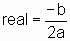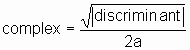In other words, take the absolute value of the discriminant before taking the square root.

Then you print the two roots using something like:

Range("B12") = FormatNumber(real,3) & "+ i*" & FormatNumber(complex, 3)

Range("B13") = FormatNumber(real,3) & "- i*" & FormatNumber(complex, 3)